Next: 3.3 The Basic Semiconductor Up: 3. The Box Integration Previous: 3.1 The Poisson Equation

Subsections

# 3.2 The Diffusion Equation

In this section, the discretization of parabolic time-variant problems is described. In its simplest representation, the right-hand side of the diffusion equation is time-invariant. Solving a diffusion problem the diffusion equation becomes time-variant. It describes the out-diffusion of matter, driven by its own concentration gradient. The diffusion flux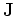can be written as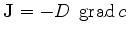(3.45)

where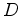denotes the diffusion coefficient and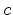is the concentration of the diffusing material.
Additionally, the conservation of material must be fulfilled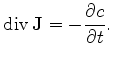(3.46)

After insertion of (3.46) in (3.45), the diffusion equation can be reformulated as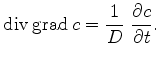(3.47)

As described in the previous section, this equation can be discretized as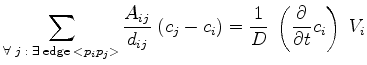(3.48)

The time derivative in this formula can be discretized by several methods. By the backward Euler method, the time derivative is approximated by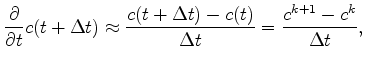(3.49)

with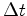the sampling interval. The discrete notation by backward Euler time discretization follows by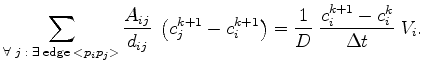(3.50)

By separating the unknowns to the left-hand side, this expression becomes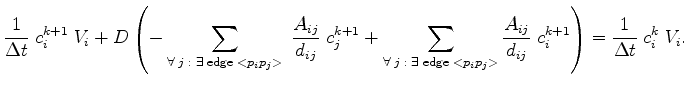(3.51)

In matrix notation, (3.51) can be written as(3.52)

with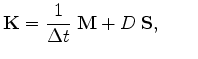(3.53)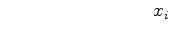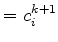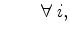(3.54)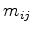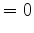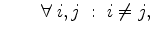(3.55)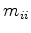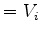(3.56)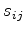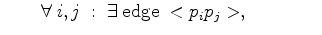(3.57)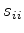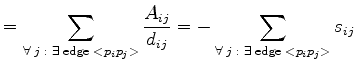(3.58)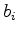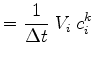(3.59)

The necessary boundary conditions for this parabolic equation can be handled as shown in the previous section. Also the requirements for an M-matrix (see Section 2.3) are satisfied. The conditions for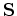can be handled as in the previous section andconsists of positive diagonal entries only. Additionally, an initial condition is required.

## 3.2.1 Initial Conditions

The initial concentration distribution at initial time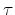is defined as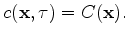(3.60)

The discrete system is satisfied by the discrete formulation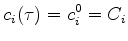for all grid points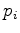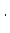(3.61)

The concentration distributions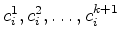can be computed by an sequential evaluation of .Next: 3.3 The Basic Semiconductor Up: 3. The Box Integration Previous: 3.1 The Poisson Equation

J. Cervenka: Three-Dimensional Mesh Generation for Device and Process Simulation